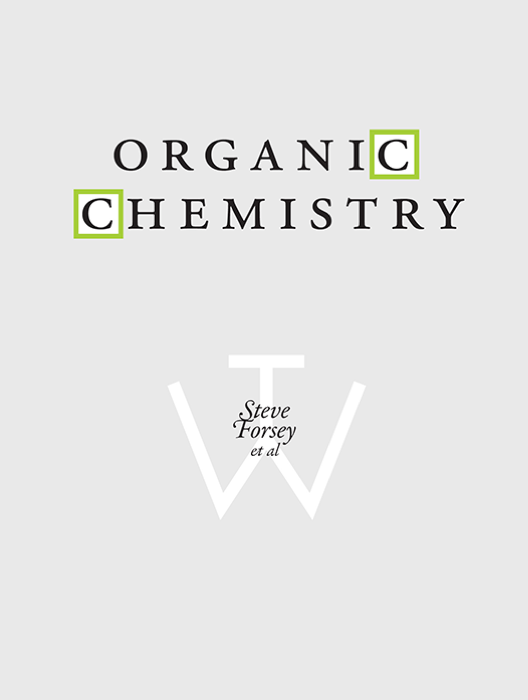# Organic Chemistry I & II

Lead Author(s): Steven Forsey

Student Price: Contact us to learn more

Top Hat Intro Course - Organic Chemistry I & II is designed for instructors who want an active, dynamic, and understandable approach to support their own efforts in the classroom. This ever-evolving textbook includes auto-graded questions, videos and approachable language in order to make difficult concepts easier to understand and implement.

## What is a Top Hat Textbook?

Top Hat has reimagined the textbook – one that is designed to improve student readership through interactivity, is updated by a community of collaborating professors with the newest information, and accessed online from anywhere, at anytime.

• Top Hat Textbooks are built full of embedded videos, interactive timelines, charts, graphs, and video lessons from the authors themselves
• High-quality and affordable, at a significant fraction in cost vs traditional publisher textbooks

## Key features in this textbook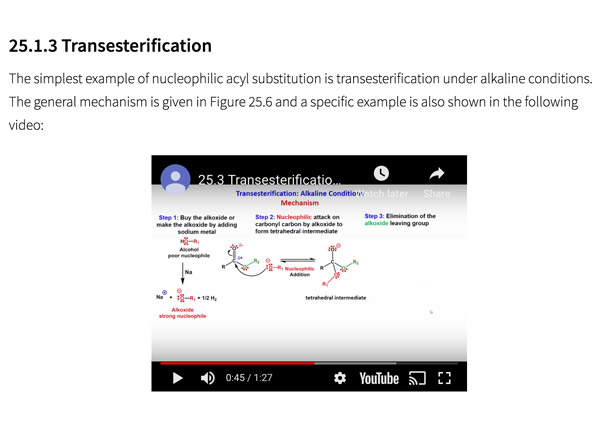Engage students with all different learning styles, offering plentiful visuals, instructional videos, interactive demonstrations, real world examples and auto-graded drawing questions.Built-in assessment questions embedded throughout chapters so students can read a little, do a little, and test themselves to see what they know! “Keeping it Real”Sections connect theory to real-world examples and demonstrate the real-life applications of Organic Chemistry.Questions are embedded right in the textbook and can be auto-graded, including chemical drawings.

## Comparison of Organic Chemistry Textbooks

Consider adding Top Hat’s Organic Chemistry textbook to your upcoming course. We’ve put together a textbook comparison to make it easy for you in your upcoming evaluation.

### Top Hat

Steven Forsey, “Organic Chemistry”, Only one edition needed

### McGraw-Hill

Carey & Giuliano, “Organic Chemistry”, 10th Edition

### Wiley

Solomons et al., “Organic Chemistry”, 12th Edition

### Wiley

David R. Klein, “Organic Chemistry”, 3rd Edition

### Pricing

Average price of textbook across most common format

#### Up to40-60%more affordable

Lifetime access on any device

#### $219 Hardcover print text only ####$301

Hardcover print text only

#### $301 Hardcover print text only ### Always up-to-date content, constantly revised by community of professors Content meets standard for Introduction to Organic Chemistry course, and is updated with the latest content ### In-Book Interactivity Includes embedded multi-media files and integrated software to enhance visual presentation of concepts directly in textbook Only available with supplementary resources at additional cost Only available with supplementary resources at additional cost Only available with supplementary resources at additional cost ### Customizable Ability to revise, adjust and adapt content to meet needs of course and instructor ### All-in-one Platform Access to additional questions, test banks, and slides available within one platform ## Pricing Average price of textbook across most common format ### Top Hat Steven Forsey, “Organic Chemistry”, Only one edition needed #### Up to40-60%more affordable Lifetime access on any device ### McGraw-Hill Carey & Giuliano, “Organic Chemistry”, 10th Edition ####$219

Hardcover print text only

### Wiley

Solomons et al., “Organic Chemistry”, 12th Edition

#### $301 Hardcover print text only ### Wiley David R. Klein, “Organic Chemistry”, 3rd Edition ####$301

Hardcover print text only

## Always up-to-date content, constantly revised by community of professors

Constantly revised and updated by a community of professors with the latest content

### Top Hat

Steven Forsey, “Organic Chemistry”, Only one edition needed

### McGraw-Hill

Carey & Giuliano, “Organic Chemistry”, 10th Edition

### Wiley

Solomons et al., “Organic Chemistry”, 12th Edition

### Wiley

David R. Klein, “Organic Chemistry”, 3rd Edition

## In-book Interactivity

Includes embedded multi-media files and integrated software to enhance visual presentation of concepts directly in textbook

### Top Hat

Steven Forsey, “Organic Chemistry”, Only one edition needed

### McGraw-Hill

Carey & Giuliano, “Organic Chemistry”, 10th Edition

### Wiley

Solomons et al., “Organic Chemistry”, 12th Edition

### Wiley

David R. Klein, “Organic Chemistry”, 3rd Edition

## Customizable

Ability to revise, adjust and adapt content to meet needs of course and instructor

### Top Hat

Steven Forsey, “Organic Chemistry”, Only one edition needed

### McGraw-Hill

Carey & Giuliano, “Organic Chemistry”, 10th Edition

### Wiley

Solomons et al., “Organic Chemistry”, 12th Edition

### Wiley

David R. Klein, “Organic Chemistry”, 3rd Edition

## All-in-one Platform

Access to additional questions, test banks, and slides available within one platform

### Top Hat

Steven Forsey, “Organic Chemistry”, Only one edition needed

### McGraw-Hill

Carey & Giuliano, “Organic Chemistry”, 10th Edition

### Wiley

Solomons et al., “Organic Chemistry”, 12th Edition

### Wiley

David R. Klein, “Organic Chemistry”, 3rd Edition

## About this textbook

### Lead Authors

#### Dr. Steven Forsey, Ph.D.University of Waterloo

Steven Forsey is currently a Professor at University of Waterloo, teaching a variety of organic chemistry courses to Chemistry, Science, Chemical Engineering, Nanotechnology and distance education students. He received his Ph.D. (2004) for Synthetic Organic Chemistry from University of Waterloo, Ontario. He is a recipient of the Excellence of Science Teaching Award and has acted as the Teaching Fellow for the Department of Chemistry since 2016.

## Explore this textbook

Read the fully unlocked textbook below, and if you’re interested in learning more, get in touch to see how you can use this textbook in your course today.

# Chapter 3: Chemical Reactivity and MechanismsThe fermentation of ethanol in wine is a multistep process that involves both elimination and addition reactions. The process involves converting one mole of glucose into two moles of ethanol, and two moles of carbon dioxide. ​

## Learning Objectives

• Identify addition, substitution, elimination and rearrangement reactions.
• Understand the two types of bond breaking and bond formation.
• Identify what type of curved arrow to use to show movement of electrons in bond formation and bond cleavage.
• Define BDE and relate the strength of bond and periodic trend to BDE.
• Understand the use of BDE in calculating enthalpy of reactions.
• Define Gibbs free energy and understand thermodynamics of a reaction.
• Understand enthalpy and entropy and be able to write the equation that relates free energy change, enthalpy change and entropy change.
• Recognize and draw the energy diagrams for an exothermic and endothermic reaction.
• Recognize and draw the energy diagram for a one-step reaction and multistep reaction.
• Understand reaction kinetics.
• Understand Hammond postulate.
• Understand the conditions for reactions under kinetic control vs. reactions under thermodynamic control.
• Recognize electrophilic and nucleophilic sites in a reaction.
• Recognize the four characteristic patterns of electron flow.
• Understand carbocation rearrangement and the order of stability of carbocations.
• Understand kinetic control vs. thermodynamic control.

## 3.1 Kinds of Organic Reactions

Organic reactions fall into four particularly broad types: substitutions, eliminations, additions, and rearrangements.

### 3.1.1. Substitution Reactions

Substitution reactions occur when an atom or a group of atoms (Br) is replaced by another atom or group of atoms (HO). In these reactions, the overall number of bonds does not change. Substitution reactions are the characteristic reactions of saturated compounds (alkanes and alkyl halides) and unsaturated compounds (aromatic compounds). For example, bromoethane reacts with sodium hydroxide to produce ethyl alcohol and sodium bromide (Figure 3.1).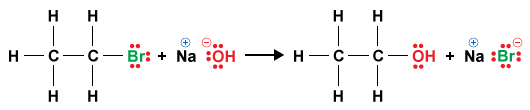Figure 3.1. A Substitution reaction.​

### 3.1.2. Elimination Reactions

Elimination reactions occur when a single reactant splits into two products. That is, two groups are eliminated from the starting material (H and Br) to form a new product (HBr). As a result of the elimination, in the starting material, two σ bonds are broken, and a π bond is formed between the adjacent atoms. These reactions give us a method for preparing compounds with double and triple bonds. For example, bromoethane undergoes dehydrobromination (loss of HBr) to form an alkene (Figure 3.2).Figure 3.2. An elimination reaction.​

### 3.1.3. Addition Reactions

Addition reactions occur when two reactants add together to form a single new product with all parts of the added reagents appearing in the product. In effect, two new atoms, or groups of atoms (H and Br, for example), are added to a starting material, a π bond is broken, and two new σ bonds are formed (Figure 3.3). In a sense, addition reactions are the opposite of elimination reactions.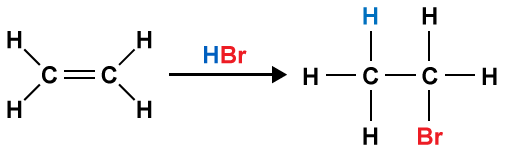Figure 3.3. An addition reaction​

### 3.1.4. Rearrangement Reactions

Rearrangement reactions occur when a single reactant undergoes reorganization of its atoms and bonds to yield a single product that is an isomer of the original reactant. The overall number of bonds does not change in rearrangement reactions. An example is the conversion of cis-2-butene into trans-2-butene by treatment with an acid catalyst (Figure 3.4).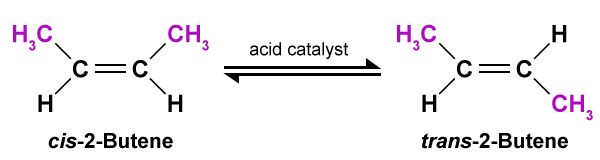Figure 3. 4. A rearrangement reaction​

Q3.1 - Level 1

Match the following (a–d) with the type of reaction.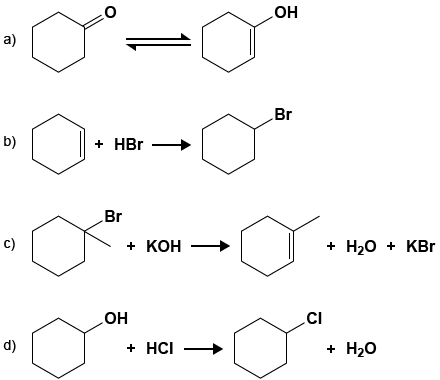Premise
Response
1

a)

A

Substitution

2

b)

B

Addition

3

c)

C

Rearrangement

4

d)

D

Elimination

Q3.2 - Level 1

Match the following (a–d) with the type of reaction.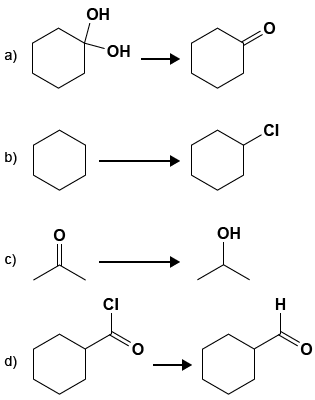Premise
Response
1

a)

A

Substitution

2

b)

B

Elimination

3

c)

C

Rearrangement

4

d)

D

Substitution

E

Addition

F

Addition

G

Rearrangement

H

Elimination

## 3.2 Bond Breaking and Bond Making

We have defined the four general kinds of reactions common in organic chemistry. Now let’s focus on how these reactions occur. A description of how a reaction occurs, that is the steps involved in the overall reaction, is called the reaction mechanism. A reaction mechanism describes how, in the course of a chemical reaction (reactants to products), bonds are broken in the reactants and formed in the products. A reaction mechanism also tells us the relative rates of each step of a chemical reaction. There are three main steps to be followed in determining a reaction mechanism:

• Design experiments that will reveal details of the chemical reaction, such as its slowest step or the specific bonds that are broken or formed during the process.
• Propose detailed mechanisms to account for the overall chemical transformation.
• Test each proposed mechanism against experimental observations and exclude those that are not consistent with the details of the chemical reaction.

Reactions of organic compounds always involve the breaking and making of covalent bonds.

### 3.2.1. Bond Cleavage

Bond cleavage requires energy (is endothermic) and may occur in two ways. Bonds may break evenly, right in the middle, leaving each side with one electron, or they may break unevenly, leaving one side with both bond electrons (with a negative charge) and the other side with an empty orbital (with a positive charge).

Asymmetrical, or heterolytic cleavage is the result of heterolysis (Figure 3.5). Heterolytic cleavage, which produces charged fragments, or ions, is described as an ionic or polar reaction.

Notice, that when the bond breaks two electrons of the broken bond are now on the leaving group (Z) which is negatively charged. The carbon is left without a full octet and has a positive charge. A positively charged carbon is called a carbocation. Heterolysis generally does not take place in isolation because both the charge stability and bond energies favor the reactants. However, elementary steps such as the one given is often the first step in a multistep reaction, since the positively charged fragment just created, is an electrophile (accepts a pair of electrons to form a new covalent bond) and the negatively charged species (negatively charged Z) can act as a nucleophile (donates a pair of electrons to form a new covalent bond). A full-headed curved arrow is used to show the movement of paired electrons in heterolysis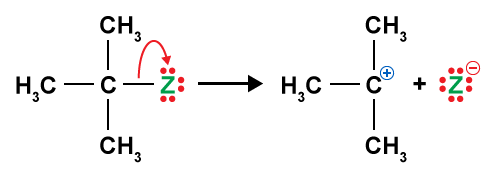Figure 3.5. Heterolytic cleavage (heterolysis).​

The other possibility is symmetrical bond cleavage through a process called homolysis, where each fragment takes away one of the bonding electrons (Figure 3.6). Homolytic cleavage produces fragments with unpaired electrons called radicals. A half-headed curved arrow is used to show the movement of each individual electron in homolysis.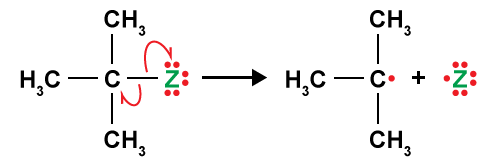Figure 3.6. Homolytic cleavage (homolysis)​

Q3.3 - Level 2

Which of the following statements is true?

A

Ionic intermediates are formed in radical reactions

B

Radicals are intermediates in polar reactions

C

Carbocations are electrophiles

D

Radicals are nucleophiles

### 3.2.2. Bond Formation

Bond formation is exothermic and occurs in two ways. A bond can form in an asymmetrical fashion whereby both bonding electrons are donated by one reactant (nucleophile) to form the new bond. This asymmetrical bond formation is called heterogenic bond formation. A full-headed curved arrow is used to show the movement of two electrons in heterogenic bond formation (Figure 3.7).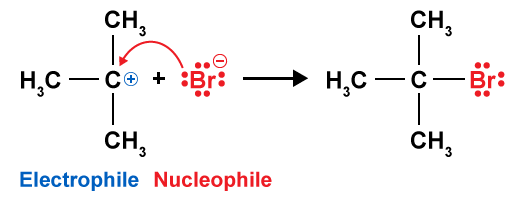Figure 3.7a. Heterogenic bond formation.

The other possibility is for a bond to be formed in a symmetrical fashion, where each reactant (radical) donates an electron to form the new covalent bond. This symmetrical bond formation is called homogenic bond formation. A half-headed curved arrow is used to show the movement of each electron in homogenic bond formation (Figure 3.8).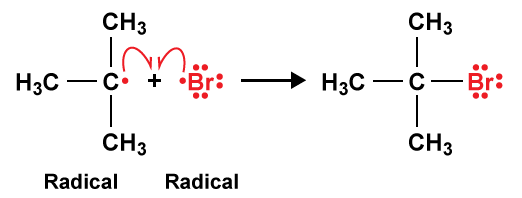Figure 3.7b. Homogenic bond formation.​

Q3.4 - Level 2

Choose the reaction equation that shows the correct use of the arrows indicating the flow of electrons to generate the indicated product.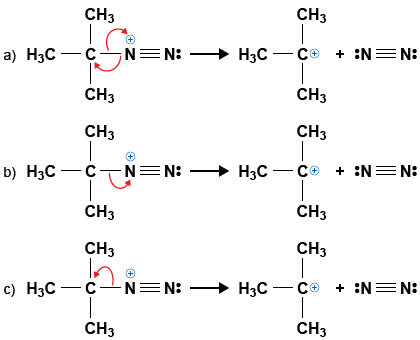A

a

B

b

C

c

Q3.5 - Level 1

Choose the reaction equation that shows the correct use of the arrows indicating the flow of electrons to generate the indicated product.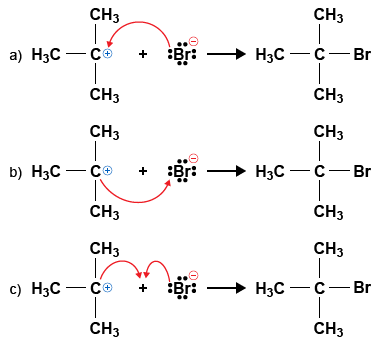A

a

B

b

C

c

## 3.3 Bond Dissociation Energy (BDE)

The energy required to homolytically cleave a covalent bond is called the bond dissociation energy (BDE, symbolized as DH° when measured under standard conditions of 1 atm and 25°C, with the compound in its standard state of gas, pure liquid, or solid).

Bond dissociation energies (BDEs) are defined as the change in enthalpy (ΔHo) for the process given below.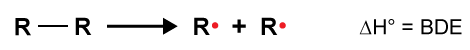Figure 3.8. Homolytic bond cleavage used for bond dissociation measurements.​​

The values obtained for the above reaction are valuable thermodynamic quantities because they correlate directly to bond strength. These values are generally determined for the gas phase reaction with all species in their standard states. However, like all thermodynamic measurements, the values obtained are temperature dependent and depend on whether they are measured in the liquid or gas phase. Table 3.1 shows the BDE (∆H°) values for some common bonds.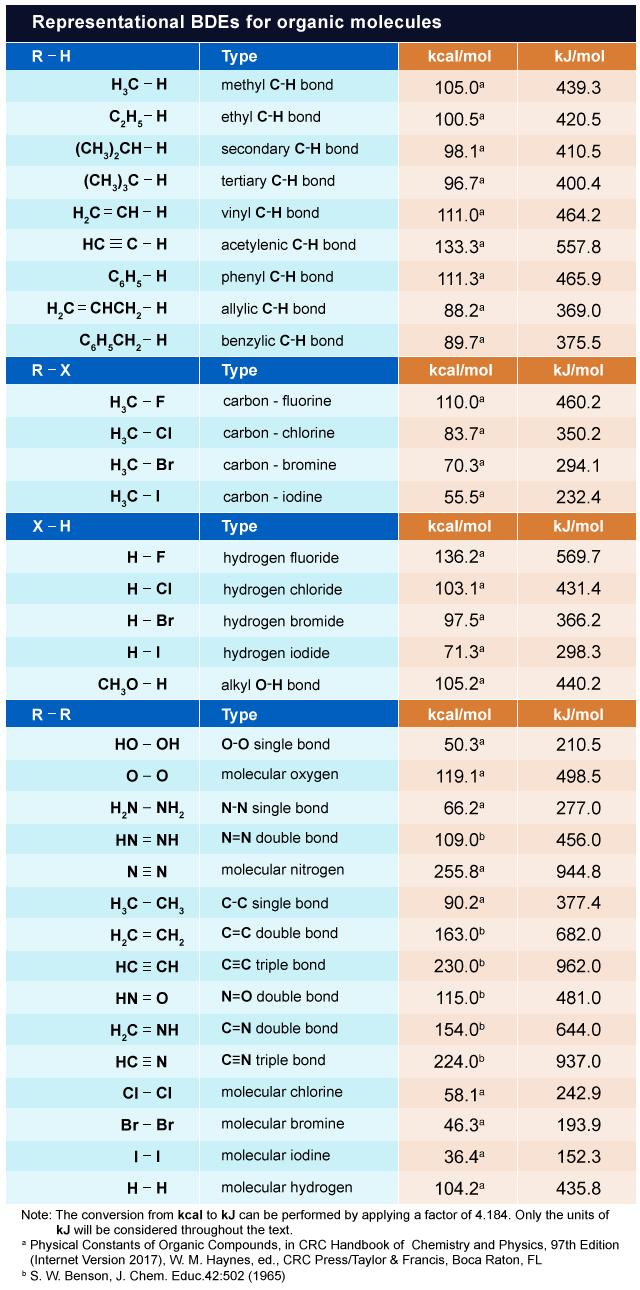Table 3.1. Representative bond dissociation energies​​

The total change in bond energy of the system, or the enthalpy (∆) for a reaction, can be calculated from the BDE by taking into consideration the energies of broken and formed bonds. This is also called the heat of reaction. Bond breaking is endothermic and requires energy (∆is positive), whereas bond formation is exothermic and releases energy (∆ is negative). The sign for the total change in enthalpy for a reaction (∆) is determined in terms of the system and not the surroundings; that is, the reaction is considered as a closed system. For a given reaction, a positive ∆ overall indicates that the system has gained energy from the surroundings, whereas a negative ∆ overall indicates that the system has lost energy to the surroundings. Figure 3.9 shows the energy diagrams for exothermic and endothermic processes.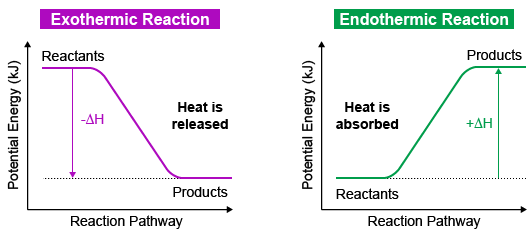Figure 3.9. Energy diagrams for exothermic and endothermic reactions

Since BDEs correlate to bond strengths, we can use Table 3.1 and Figure 3.9 to derive some generalizations:

• A stronger bond has a higher BDE because more energy is required to break it.
• Starting from the same compound, more energy must be supplied to produce a less stable radical than to produce a more stable radical. This is because a less stable radical has greater potential energy.
• As bond length increases (going down a group of the periodic table, for example), the bond becomes weaker and its BDE decreases.
• If a reaction is endothermic (Δ > 0), then bonds broken in its reactants are stronger than bonds formed in its products.
• If a reaction is exothermic (Δ < 0), then bonds formed in its products are stronger than bonds broken in its reactants.
• The overall enthalpy change for a reaction (Δrxn) is given below (Equation 3.1):Equation 3.1. Calculating the enthalpy change for a reaction using BDE​

Example: To demonstrate how Equation 3.1 can be used to calculate BDE, consider the substitution reaction between propane and Cl2 to give 2-chloropropane and H-Cl. Using values of BDE from Table 3.1 and Appendix 22: Bond Dissociation Energies, calculate the magnitude and sign of ΔHo for the reaction.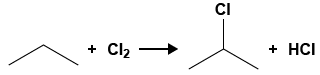To figure out the answer, think about all of the bonds involved in the reaction

Solution: To calculate the magnitude and sign of ΔHo for the reaction, we have to look up the BDE for each bond broken and formed in the reaction from Table 3.1. We also have to remember that bond breaking is endothermic (positive value of BDE) and bond formation is exothermic (negative value of BDE).Determine bonds broken and new bonds formed

Bonds broken and corresponding BDEs
Bond                                        BDE
H-CH(CH3)2                          + 411 kJ/mol
Cl-Cl                                        + 243 kJ/mol

Bonds formed and corresponding BDEs
Bond                                        BDE
Cl-CH(CH3)2                          + 355 kJ/mol
H-Cl                                          + 431 kJ/mol

Calculate:

The overall reaction is exothermic; thus, the bonds formed in the products are stronger than bonds broken in the reactants.

Q3.6 - Level 1

Which of the following statements is true?

A

Bond dissociation energies increase down a column of the periodic table

B

When ΔH° is positive, more energy is released in forming bonds than is needed to break bonds

C

When ΔH° is negative, more energy is needed to break bonds than is released in forming bonds

D

Bond dissociation energies decrease down a column of the periodic table

Q3.7 - Level 2

Using Table 3.1, rank each of the indicated bonds in order of increasing BDE.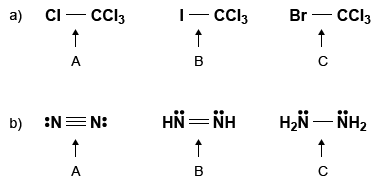A

2

B

1

C

3

3D Molecule*: nitrogen

3D Molecule*: diazene

3D Molecule*: hydrazine

Q3.8 - Level 1

Using Table 3.1, rank each of the indicated bonds in order of increasing BDE.A

1

B

2

C

3

### 3.4 Thermodynamics

The thermodynamics of a reaction is based on the difference in free energy between reactants and products. The extent that reactants in a given reaction are converted to products is determined by the difference in their free energy ∆ (Gibbs free energy change). Consider the reaction of A and B to give C and D (Figure 3.10). This reaction exhibits a negative ∆ and is, therefore, spontaneous. However, starting from A and B, an equilibrium mixture of A, B, C, and D is obtained because the reverse reaction is possible and an equilibrium is established.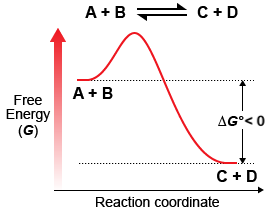Figure 3.10. Energy diagram of an exergonic process in which reactants are converted to products.

Figure 3.10 depicts a situation in which we have one molecule of A reacting with one molecule of B (reactants, R). What will happen if we have a large number of molecules of A and B? Imagine we are dealing with several moles of A and several moles of B, and we want to evaluate the effect of changing concentrations on the value of ∆. At the start of the reaction, we only have A and B, and ∆ is large because the concentrations of A and B are large. However, as the reaction progresses, the concentrations of A and B decrease while the concentrations of C and D increase. The changing concentrations result in changes in the value of ∆. The free energy decreases as the reaction progresses until a minimum has been reached, at which point an equilibrium is established where the concentrations of the reactants and products remain constant. Specific concentrations of reactants and products can be determined for any given reaction and the exact position of equilibrium is described by the equilibrium constant, Keq (Equation 3.2).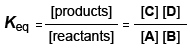Equation 3.2. Equilibrium constant expression for a reaction of A and B to give C and D

By definition, Keq is the equilibrium concentrations of products divided by the equilibrium concentrations of reactants. The exact position of equilibrium is represented by Keq and this term is related to the change in free energy (∆) as follows (Equation 3.3):Equation 3.3. Relationship between free energy change and equilibrium constant

where:

• Keq = [product] / [reactant] at equilibrium
• R = 8.314 J × K-1 × mol-1 or 1.987 cal × K-1 × mol-1
• T = temperature in Kelvin (K)

From Equation 3.3, we can make the following generalizations:

• determines the maximum yield of products.
• Equilibrium always favors the more stable species or species lower in energy.
• If ∆< 0, then the products will be favored at equilibrium (Keq > 1). The energy of the products is lower than the energy of the reactants. Such processes are spontaneous and are called exergonic.
• If ∆ > 0, then the reactants will be favored at equilibrium (Keq < 1). The energy of the products is higher than the energy of the reactants. Such processes are not spontaneous and are called endergonic.

Q3.9 - Level 1

Given the data K$_{eq}$ = 2.7, determine if the reaction favors reactants or products.

A

Favors reactants

B

Favors products

C

Cannot be determined

Q3.10 - Level 1

Given the data ∆G° = +1.49 kJ/mol, determine if the reaction favors reactants or products.

A

Favors reactants

B

Favors products

C

Cannot be determined

Q3.11 - Level 2

Given the data K$_{eq}$ = 0.6, determine if the reaction favors reactants or products.

A

Favors reactants

B

Favors products

C

Cannot be determined

## 3.5 Enthalpy and Entropy

In the previous section we described spontaneous and non-spontaneous processes using Gibbs free energy. If you recall the second law of thermodynamics from your general chemistry course, then you will know of the relationship between Gibbs free energy (Δ), enthalpy change (Δ), and entropy change (Δ) as shown in the equation below (Equation 3.4):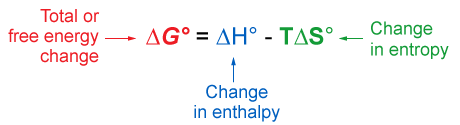Equation 3.4. Relationship between Gibbs free energy, enthalpy change, and entropy change.

In Equation 3.4, T is the absolute temperature in Kelvin. The change in entropy (Δ) represents the change in molecular disorder or randomness. This relates to the molecules’ internal vibrational motion (vibrational energy) and their ability to move through the system (translational energy), rotate, and tumble (rotational energy). Entropy changes are very important when looking at changes in chemical structure, such as cyclization reactions, conformational changes, or mixing of solutions; however, for many reactions Δ is small and is often assumed to be negligible for reactions performed in solution.

For chemical processes where the entropy change is small (~0), the enthalpy change is essentially the same as the change in Gibbs free energy. Energy diagrams for these processes will often plot the potential energy instead of free energy, for simplicity.

In Equation 3.4 above, the first term (Δ) is associated with the change in enthalpy of the surroundings. The second term (T Δ) is associated with the change in entropy of the system. Therefore, Δ is a good approximation of an expression representing total enthalpy. Because the Δ term is often much larger than the T Δterm, the sign of Δ will be determined by the sign of Δ. From Equation 3.4 and our knowledge of general chemistry, we can come up with the following generalizations:

• A spontaneous reaction will occur if the process gives off heat (Δ is negative, exothermic) and becomes more disordered (Δis positive)
• A spontaneous reaction will not occur if the process requires an increase in enthalpy (Δ is positive, endothermic) and an increase in order (Δ is negative)
• The questionable cases are those in which the entropy and enthalpy factors work in opposition. In general, the Δ factor dominates at low temperature and the T Δterm dominates at high temperatures
• When Δ> 0, the reaction is favored due to an increase in entropy
• When a single starting material forms two products, Δ > 0 (increase in disorder)
• When a cyclic molecule is converted to an acyclic one, Δ > 0 (increase in disorder)
• When Δ < 0, the reaction is not favored due to the decrease in entropy, unless the process has a large negative Δ value
• Conditions that favor reactants: Keq < 1, ΔH° > 0, ΔG° > 0, ΔS° < 0
• Conditions that favor products: Keq > 1, ΔH° < 0, ΔG° < 0, ΔS° > 0

Q3.12 - Level 2

For a reaction carried out at 298 K and 1 atm and for which ΔH° = +30 kJ/mol and ΔS° = +153 J/mol, determine if the reaction favors reactants or products.

A

Favors reactants

B

Favors products

C

Cannot be determined

Q3.13 - Level 1

For an exothermic reaction with a positive value of ΔS°$_{sys}$, determine if the reaction favors reactants or products.

A

Favors reactants

B

Favors products

C

Cannot be determined

Q3.14 - Level 1

For an endothermic reaction with a negative value of ΔS°$_{sys}$, determine if the reaction favors reactants or products.

A

Favors reactants

B

Favors products

C

Cannot be determined

## 3.6 Energy Diagrams

Energy diagrams are used to depict the energy changes that occur during a reaction. The vertical axis of the energy diagram represents the total energy of all reactants and the horizontal axis represents the progress of the reaction from start (left) to finish (right).

At the start of a reaction between two species (A and B, for example), both species have the total amount of energy indicated by the reactant level on the left side of the reaction energy diagram. As the two species come together, their electron clouds repel each other, causing an increase in the energy level. Despite the repulsion caused by electron clouds, collisions with sufficient energy and proper orientation will force the species to approach each other until bonds start to break and form. This continues until a structure of maximum energy is attained, which is called the transition state. At the transition state, bond breaking and bond making occur, sometimes to the same extent and at other times to a different extent. The transition state cannot be directly observed or isolated. However, it is thought of as an activated complex of reactants in which the old bonds are partially broken and the new bonds of the product are partially formed (Figure 3.11). The speed of a reaction on an energy diagram is associated with the difference in energy between the reactants and transition state, which is called the free energy of activation (ΔG).

A reaction energy diagram is drawn differently depending on whether the reaction is exergonic or endergonic.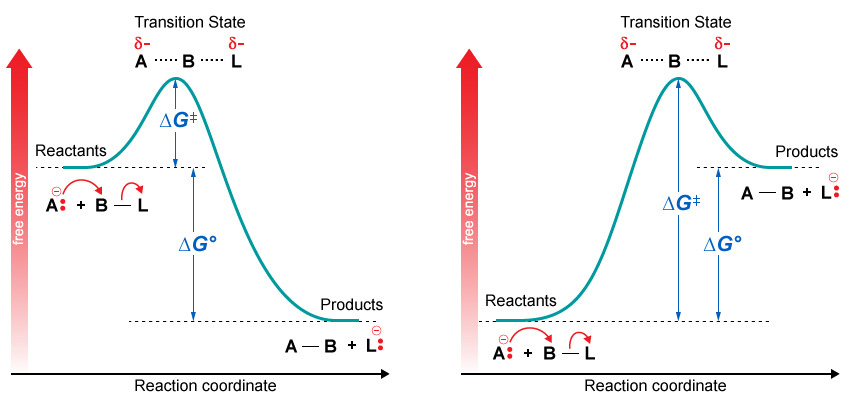Figure 3.11. Free energy diagrams for exergonic (left panel) and endergonic (right panel) reactions.

As stated above, when there is a small change in entropy of the system (Δ), Δ is approximately equal to Δ.

We now talk about the difference in the potential energy between the reactants and products. The free energy of activation is now called the activation energy (Ea) because we are now neglecting the change in entropy of the system (Figure 3.12).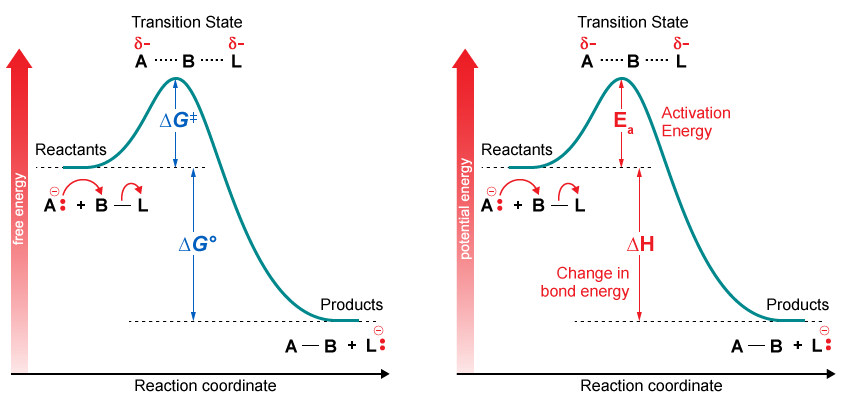Figure 3.12. Free energy and potential energy diagrams for an exergonic reaction.

What does the potential energy of a molecule mean? All molecules possess internal strain caused by conformational distortion, or non-optimal bonding with respect to a reference compound. This internal strain represents energy that is stored within the molecule. Thus, molecules have potential energy (PE) or stored energy that can be released under the right reaction conditions. The most common analogy is a compressed or stretched spring that is under stress and thus has potential (stored) energy stored (PE). The stressed spring has higher potential energy than in the relaxed state and, therefore, has the potential to do work, such as moving a block when released. Similarly, molecules can do work via chemical reactions as demonstrated by the force created by the decomposition of sodium azide (NaN3) with an electrical spark to produce sodium metal and nitrogen gas in car airbags.

### 3.6.1. One-step Reaction Mechanism

Consider a bimolecular nucleophilic substitution reaction (SN2) where the two reactants (OH and CH3Br) are converted into two products (CH3OH and Br). The reaction goes through a concerted one-step process in which bond breaking and bond forming occur simultaneously (transition state). The diagram below is the energy diagram for a one-step exothermic reaction. It shows how the energy of the system changes during the chemical reaction (Figure 3.13).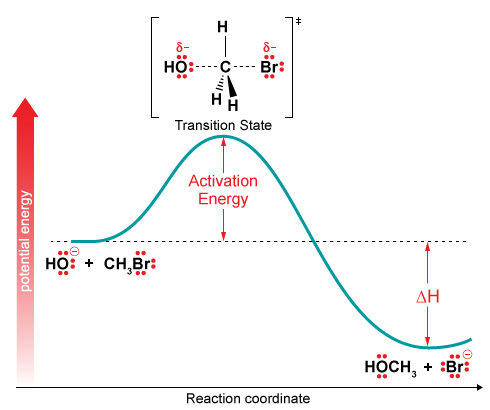Figure 3.13. Reaction energy diagram for a one-step exothermic reaction.

The products are at a lower energy than the reactants. Because this is an exothermic reaction, heat is given off, and the reaction should be favorable from an energy standpoint. The energy difference between the reactants and the products is H in the diagram. The amount of energy required by reactants to convert to products is the activation energy Ea (Eact). At the start of the reaction, the energy of the system is the same as the energy of the reactants.

As the reaction progresses to the transition state (the point of highest energy between reactants and products), energy must be added to the system. After the transition state is attained, the energy of the system decreases as the high-energy structure turns to product. The final energy of the system is the energy of B. An amount of energy equal to the activation energy Ea must be added to the system or no reaction will occur. Energy equal to ∆H is released from the system as the products are formed.

### 3.6.2. Two-step Reaction Mechanism

Now, let’s consider a two-step reaction mechanism, such as an SN1 reaction between chloride and tert-butyl bromide. The energy diagram looks somewhat different (Figure 3.14).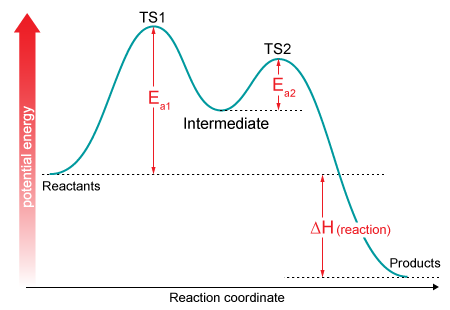Figure 3.14a. Reaction energy diagram for a two-step exothermic reactionFigure 3.14b. Reaction mechanism for a two-step exothermic reaction​

Given that there are two steps involved, there are also two transition states and two activation energies to consider, as well as the carbocation intermediate. The first, bond-breaking step from the reactants to the intermediate is an endothermic process, because the carbocation is significantly less stable (higher in energy) than the starting alkyl halide. The transition state for this step, labeled TS1, can be thought of as the point at which the carbon–chlorine bond is half-way broken. The second step, an attack on the carbocation electrophile by the chloride nucleophile and formation of the new carbon–chlorine bond, is a highly exothermic step, which passes through a second and much lower energy transition state, labeled TS2. The intermediate is, therefore, depicted as an energy “valley” situated between TS1 and TS2.

Q3.15 - Level 3

Use the following diagram to answer the question below.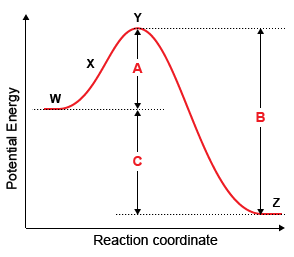Premise
Response
1

Which letter corresponds to the activation energy of the reaction?

A

W

2

Which letter corresponds to the change in energy for the overall reaction?

B

X

3

Which letter corresponds to the activation energy for the reverse reaction?

C

C

4

Which letter corresponds to the transition state?

D

A

E

Y

F

Z

G

B

Q3.16 - Level 1

How many transition states are present in the reaction in the energy diagram?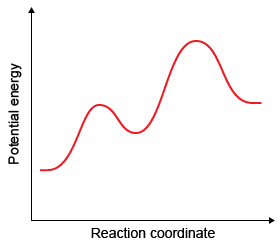A

0

B

1

C

2

D

3

Q3.17 - Level 1

What is a transition state?

A

An intermediate in a reaction that can be isolated

B

The reaction starting materials

C

A local maximum on the energy diagram

D

A local minimum on the energy diagram

Q3.18 - Level 2

What is an intermediate?

A

A local maximum on the energy diagram

B

A point on the reaction pathway that has a discrete lifetime

C

A point that is half-way between the starting materials and products

D

The highest energy compound on an energy diagram

Q3.19 - Level 1

Click on the circle that represents TS$_1$.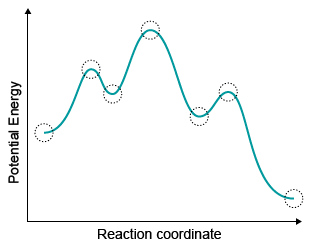Q3.20 - Level 2

Click on the circle that represents the first intermediate.## 3.7 Kinetics

In the previous sections we have been discussing thermodynamics, which deals with how energy is distributed under the influence of entropy. Referring to the difference in the relative energies of the reactants and products, thermodynamics will tell us if the reaction will work, but not how fast the reaction will go. Specifically, in thermodynamics, the difference in free energy determines the yield of the product that can be expected from the chemical reaction; however, to determine how fast the reaction will occur, we must discuss the rate of the reaction, or its reaction kinetics.

The rate (speed) of reactions can be estimated by looking at the height of the energy barrier (relative to the reactants) in their energy diagrams. The higher the energy barrier the slower the rate of reaction; conversely, the lower the barrier, the faster the rate. To help you visualize the energy involved in the potential energy reaction coordinate diagram, imagine yourself as a mountain climber who is moving along the reaction path from left to right. To get to the bottom of the mountain on the right side you must climb up the hill with a height Ea.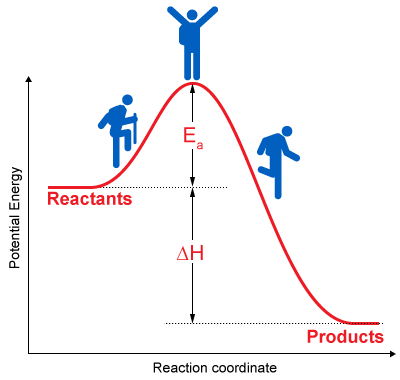Figure 3.15​. Illustration of the analogy of a mountain climber moving along the reaction path in a potential energy diagram.

### 3.7.1. Activation Energy

The activation energy (Ea or Eact) is the energy difference between reactants and the transition state (TS). It is the energy barrier that must be overcome by reactants to form products. A slow reaction has a larger activation energy while a fast reaction has a smaller activation energy (Figure 3.16).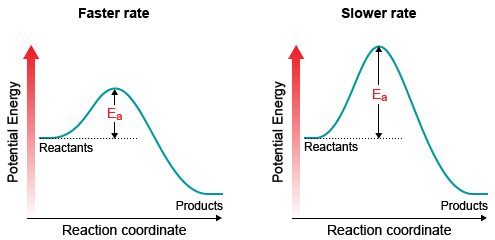Figure 3.16. Comparing two exothermic reactions (faster and slower) with different activation energies.

Looking at Figure 3.16, there is a very important concept expressed that relates to the kinetics of the reaction. The activation energy for the slower reaction is much higher than the activation energy for the faster reaction. This should make intuitive sense because the slower reaction has a high activation barrier (high Eact). It requires a great deal of added energy for the reaction to take place. The faster reaction has a much lower activation barrier. Only a small amount of energy is required. For a multistep reaction, the slow step is called the “rate-limiting step” or the “rate-determining step”. Other factors that affect reaction rates are concentration, temperature, and steric hindrance.

• Concentration: The higher the concentration (for reactants involved in the rate-determining step), the faster the rate. Increasing the concentration increases the chances for collisions between reacting molecules, which increases rate.
• Temperature: The higher the temperature, the faster the rate of reaction. An increase in temperature increases the average kinetic energy of reacting molecules and the fraction of molecules that have enough kinetic energy to overcome the energy barrier. As a rule of thumb, increasing the temperature by 10°C doubles the reaction rate.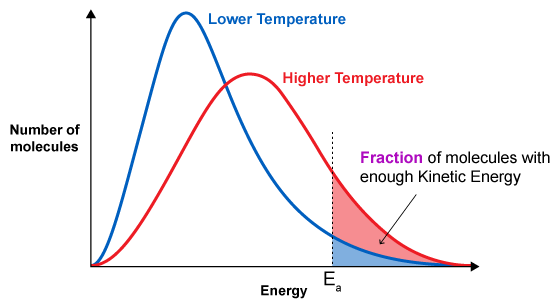Figure 3.17​. Distribution of molecular energy. The areas shown in blue or red after the dash represent the fraction of molecules that have sufficient energy to undergo a reaction.
• Steric hindrance: The geometry of the reactants and the orientation of collisions may have an impact on the rate of reaction. For example: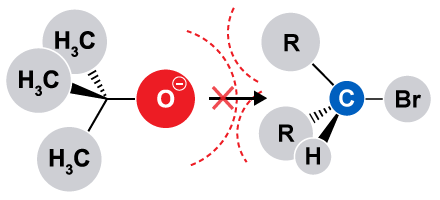Figure 3.18. Illustration of steric interaction caused by electron repulsion between the nucleophile (tert-butoxide) and the electrophile (alkyl bromide).
• Thermodynamic quantities such as Δ, Δ, and Keq do not affect reaction rate.

### 3.7.2. Rate Equation

The rate equation or rate law is an equation that shows the relationship between the rate of a reaction and the concentration of its reactants.

Rate equation for a multi-step reaction: The following is the general reaction mechanism for an SN1 reaction that occurs in two steps.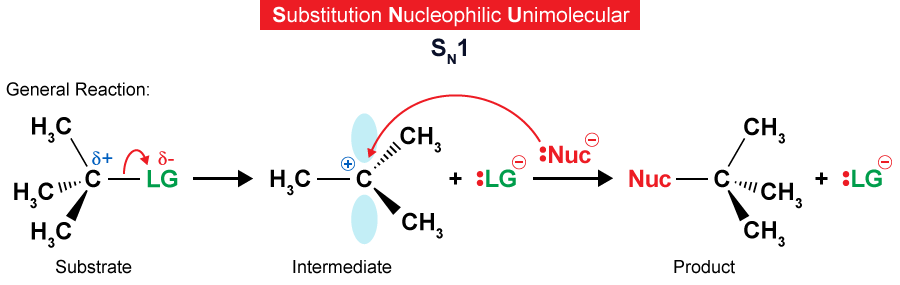Figure 3.19.

The reaction coordinate diagram for an SN1 reaction was previously shown (see Figure 3.14).

SN1 reactions have first-order kinetics; that is, their rates depend on the concentration of the substrate [substrate] to the power of one as follows:

rate = k[substrate]                      k in this equation is rate constant

In this equation, if the concentration of the substrate is doubled, then the rate of the reaction will also double. Doubling the concentration of the nucleophile, however, will have no effect on the rate because the nucleophile does not participate in the rate-determining step and, therefore, its concentration does not appear in the rate equation. The nucleophile only participates in the fast, non-rate-determining step.

Rate equation for a one-step reaction or concerted reaction, such as the SN2 reaction: An SN2 reaction has second-order kinetics; that is, the rate depends on the concentrations of the substrate ([substrate]) and nucleophile ([nucleophile]).